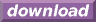#Calcute: freeware calculator

## Hyperbolic Sine (sinh)

Calcute implements the hyperbolic sine function with the sinh function.

```sinh(1)
1.17520119364
```

It is defined in terms of the exponential function as sinh(x) = (exp(x) - exp(-x)) / 2.

A similarity exists between the trigonometric sine and the hyperbolic sine functions. While the former is best described graphically using a unit circle with equation (x2 + y2) = 1, the hyperbolic sine follows the unit hyperbola with equation (x2 - y2) = 1 instead.